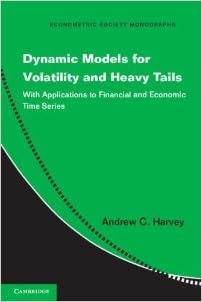## Sunday, March 23, 2014

### GAS and DCS Models: Tasty Stuff, and I'm Hungry for MoreGeneralized Autoregressive Score (GAS) models, also known as Dynamic Conditional Score (DCS) models, are an important development. They extend significantly the scope of observation-driven models, with their simple closed-form likelihoods, in contrast to parameter-driven models whose estimation and inference require heavy simulation.

Many talented people are pushing things forward. Most notable are the Amsterdam group (Siem Jan Koopman et al.; see the GAS site) and the Cambridge group (Andrew Harvey et al., see Andrew's interesting new book). The GAS site is very informative, with background description, a catalog of GAS papers, code in Ox and R, conference information, etc. The key paper is Creal, Koopman and Lucas (2008). (It was eventually published in 2012 in Journal of Applied Econometrics, proving once again that the better the paper, the longer it takes to publish.)

The GAS idea is simple. Just use a conditional observation density $$p(y_t |f_t)$$ whose time-varying parameter $$f_t$$ follows the recursion
\begin{equation}f_{t+1} = ω + β f_t + α S(f_t) \left [ \frac{∂logp(y_t | f_t)}{∂ f_t} \right ],~~~~~~~(1) \end{equation} where $$S(f_t)$$ is a scaling function. Note in particular that the scaled score drives $$f_t$$. The resulting GAS models retain observation-driven simplicity yet are quite flexible. In the volatility context, for example, GAS can be significantly more flexible than GARCH, as Harvey emphasizes.

Well, the GAS idea seems simple. At least it's simple to implement if taken at face value. But I'm not sure that I understand it fully. In particular, I'm hungry for a theorem that tells me in what sense (1) is the "right" thing to do. That is, I can imagine other ways of updating $$f_t$$, so why should I necessarily adopt (1)? It would be great, for example, if (1) were the provably unique solution to an optimal approximation problem for non-linear non-Gaussian state space models. Is it? (It sure looks like a first-order approximation to something.) And if so, might we want to acknowledge that in doing the econometrics, instead of treating (1) as if it were the DGP? And could we somehow improve the approximation?

To the best of my knowledge, the GAS/DCS literature is silent on such fundamental issues. But based on my experience with the fine scholarship of Creal, Harvey, Koopman, Lucas, and their co-authors and students, I predict that answers will arrive soon.